Welcome to part-12 of the series and the part on Algorithm running time questions.

A lot of time, the interviewer will ask you to write an algorithm and then ask you the Big O or worst running time of it. This part will teach you how to deal with questions like them.

Question 72- What is the Big O of the below simple for loop?

``````for(var i=1; i<=n; i++) {
a = a + b;
}
``````

Answer-The loop will run for “n” times and “a+b” can be considered as constant “c”. So, the Big O or worst running time will be “c.n”. No, we can ignore the constant as it will be very small with large “n”.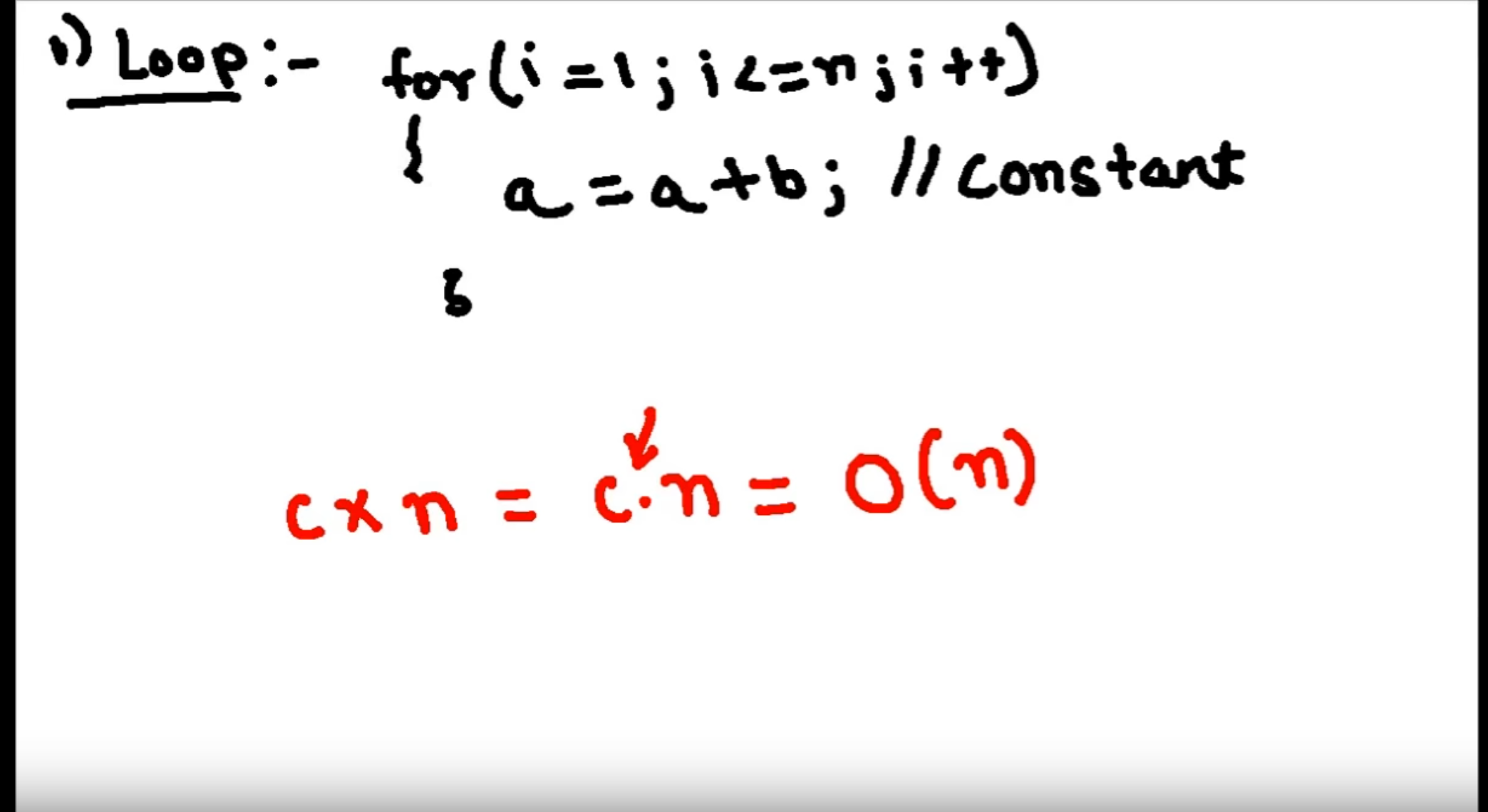Simple Loop

So, the Big O of a single loop is O(n).

Question 73- What is the Big O of the below nested for loop?

``````for(var i=1; i<=n; i++) {
for(var j =1; j<=n; j++) {
a= a + b;
}
}
``````

Answer-The worst running time or Big O of any nested for loop is calculated as below. The outer “for” loop will run for “n” times and the inner “for” loop will run for another “n” times. And the constant will be multiplied with it. But for larger “n” we can ignore the “c”. The Big O is O(n²).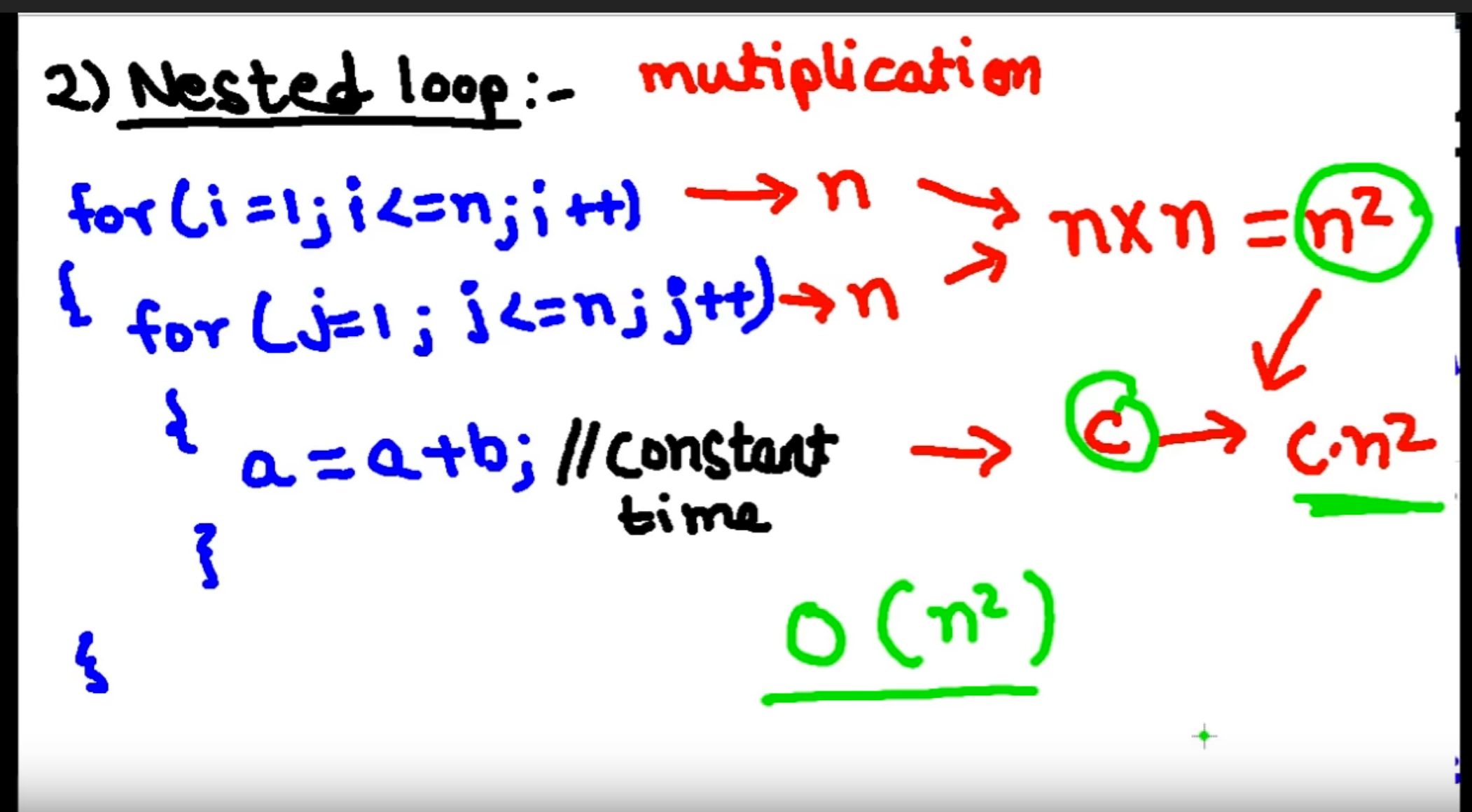Nested Loop

Question 74- What is the Big O of the below consecutive statements containing for loops?

``````a = a + b;

for(var i=1; i<=n; i++) {
x = x + y;
}

for(var j =1; j<=n; j++) {
c = c + d;
}
``````

Answer-We have three consecutive statements here. One is a Constant and two for loops, which are after each another. IN such cases we do addition of all.

As you can see below diagram. The first constant statement running time can be taken as “c1”, the second for loop will be “c2.n” and the third for loop will be “c3.n”.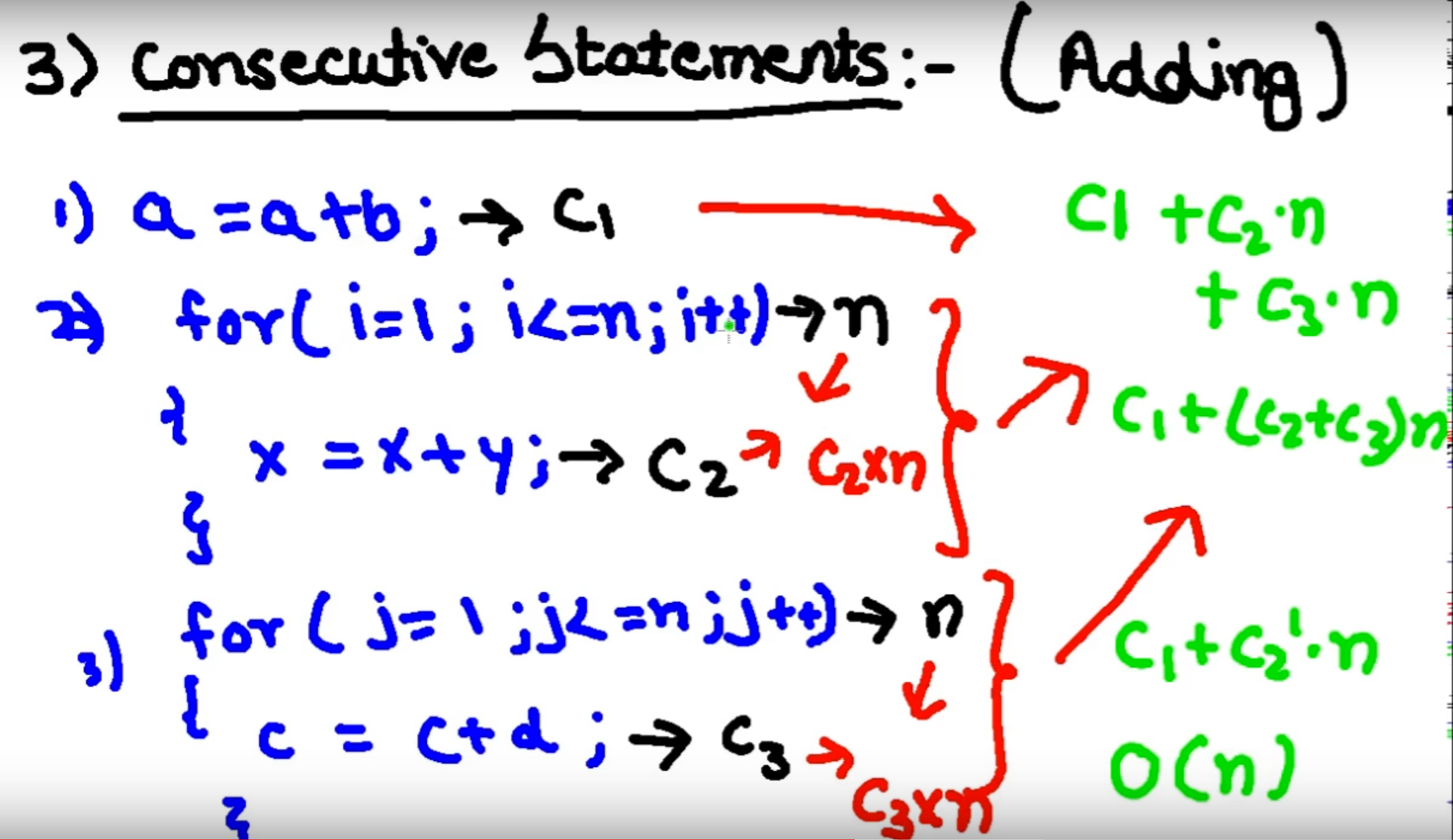Consecutive statements

We will then add all of them as c1 + c2.n + c3.n Then we are taking out the common as c1 + (c2+c3)n

We can substitute “c2+c3” with a new constant “c2 dash”. Now, we know that we can ignore the constant. So, the Big O of the consecutive statement is O(n).

Question 75- What is the Big O of the below if-else statements containing for loops?

``````if(true) {
for(var i=1; i<=n; i++) {
x = x + y;
}
} else {
for(var i=1; i<=n; i++) {
for(var j =1; j<=n; j++) {
a= a + b;
}
}
}
``````

Answer-For situations like above where we have a if-else statement, we take the running time of the part which produces the worst running time.

Like the “for loop” inside “if” statement will take O(n) and the “nested for loop” will take O(n²). Now, we take the worst case of the if-else which is O(n²).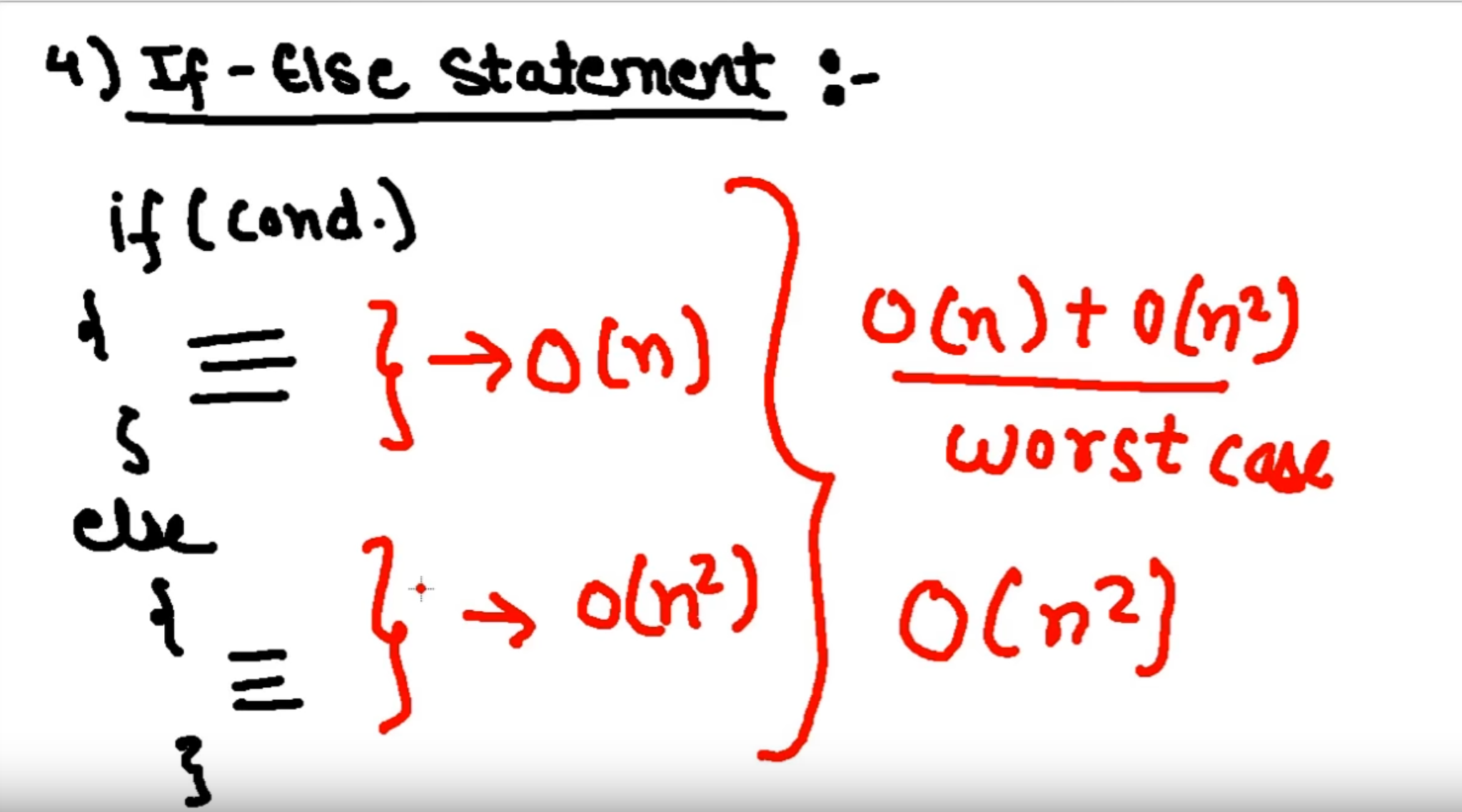if-else statement

So, the Big O of the the mentioned if-else statement is O(n²).

Question 76- What is the Big O of the below for loop in which value of “i” increases logarithmically?

``````for(var i=1; i<=n; i*=2) {
...
...
...
}
``````

Answer-The above for loop, the value of “i” increases logarithmically and every-time it will increase 2 times. So, if we give n=32, then the “for loop” will run 1, 2, 4, 8, 16, 32

So, the loop will run for “log n” instead of “n” times. So, the running time of the algorithm will be O(log n)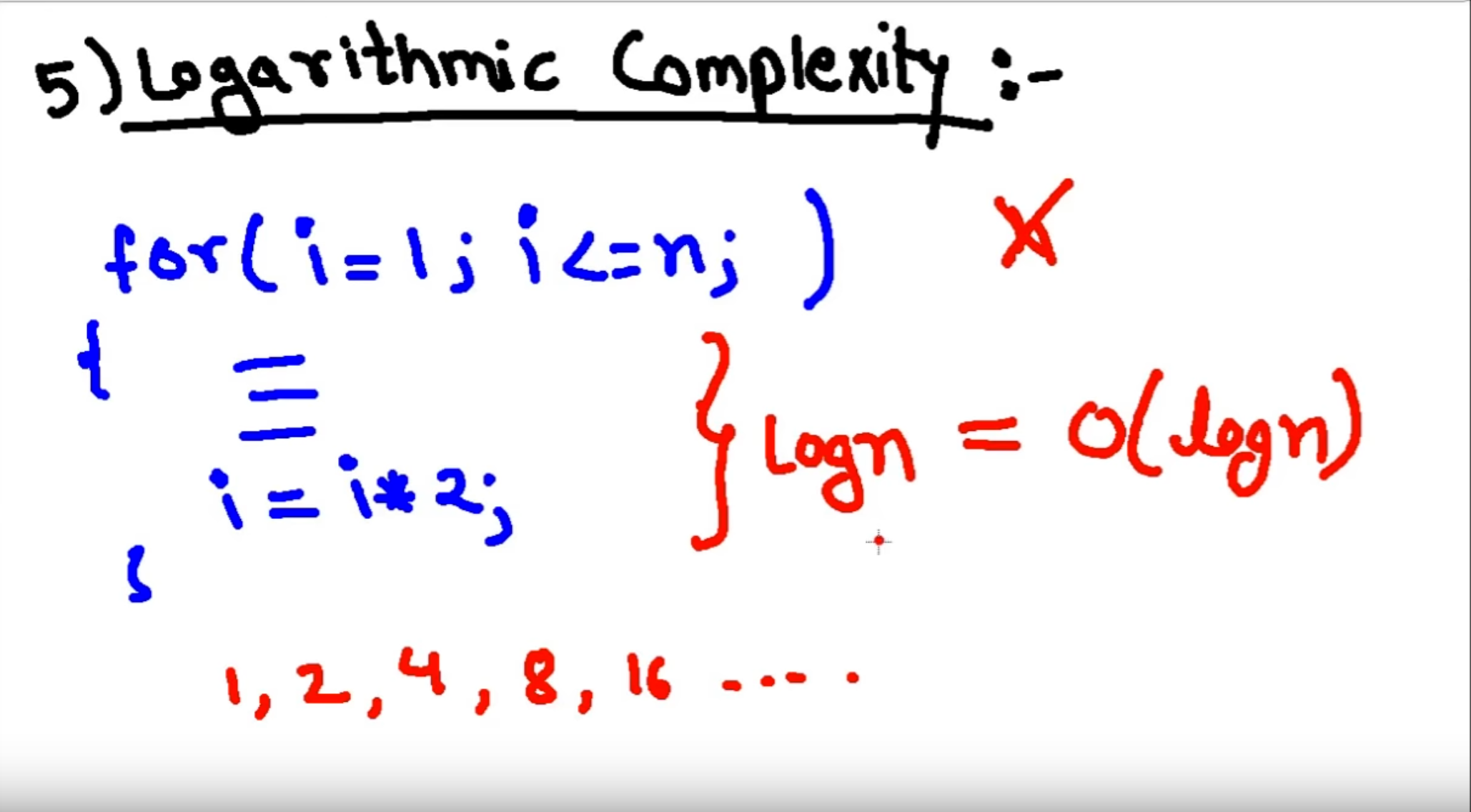logarithmically

Question 77- What is order of time complexity or Big O? Answer- The order of Big O is `O(1) < O(log n) < O(n) < O(n log n) < O(n²)`

So, we should try to avoid algorithm with O(n²)running time. They are generally the algorithms with “nested for loops”.

Question 78- What is the difference between the three Asymptotic Notations O , Ω and Θ? Answer-Asymptotic analysis of an algorithm refers to defining the mathematical boundation of its run-time performance. Using asymptotic analysis, we can very well conclude the best case, average case and worst case scenario of an algorithm.

• Worst Case- Maximum time required for program execution. It is called “Big Oh” notation and denoted by O.

• **Best Case **— Minimum time required for program execution. It is called “Omega” notation and denoted by Ω.

• Average Case — Average time required for program execution. It is called “Theta” notation and denoted by Θ.

“Big Oh” notation, O The notation O(n) is the formal way to express the upper bound of an algorithm’s running time. It measures the worst time complexity or the longest amount of time an algorithm can possibly take to complete. It is written mathematically as below-

``````O(g(n) = {f(n) : there exists c>0 and n0 such that f(n)<c.g(n) for all n > n0 }
``````

We will now see the graph for “Big Oh” to understand the above mathematical term. As in the below diagram, suppose the f(n) = 5n² + 2n + 1. So, the g(n) will be , as it is the upper bound and we will ignore the constant. Now, for n=1, f(n) will be 8 and g(n) will be 1. To make g(n) equal to f(n) we multiply the constant 8 to it. Now, f(n) = c.g(n). Next, we will take n=2, f(n) will be 25 and c.g(n) will be 32. If we plot a graph for the same, the c.g(n) will intersect with f(n) when n=1 and after that it will always be grater then it till infinity. So, the upper bound is O(n²) which is also the worst case scenario.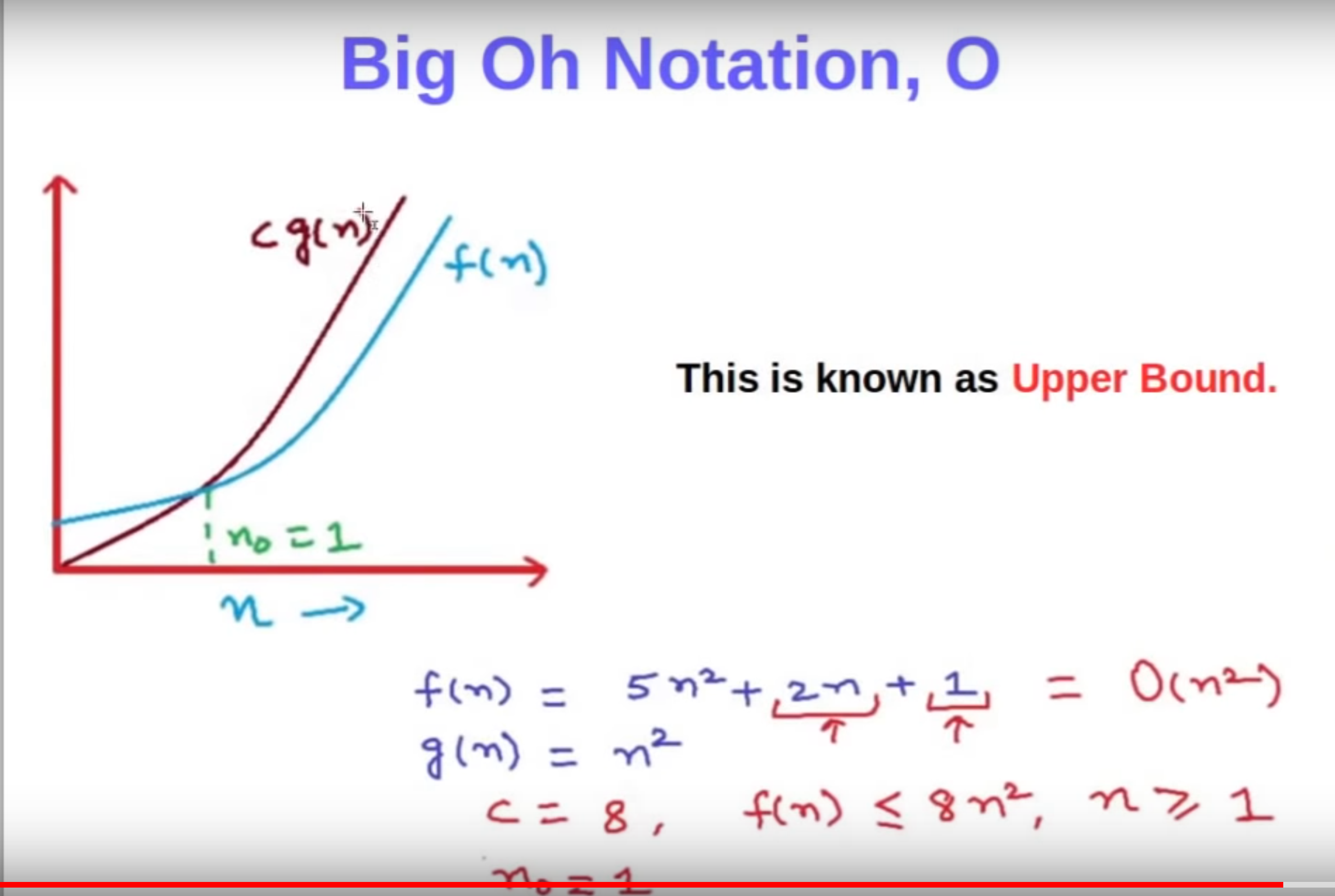Big Oh

“Omega” notation, Ω The notation Ω(n) is a formal way to express the lower bound of an algorithm’s running time. It measures the best case time complexity. It is written mathematically as below-

``````Ω(g(n) = {f(n) : there exists c>0 and n0 such that f(n)>=c.g(n) for all n >= n0 }
``````

We will consider the below diagram to understand it. Suppose the f(n) = 5n² + 2n + 1 and the g(n)=n². Now, the condition is that “f(n) should always be greater then or equal to c.g(n)”. We will multiply constant 5 to g(n), because if we multiply 5 then also the f(n) will have 2n +1 which will always make it greater then c.g(n) For n=0 and c=5, f(n) will be 1 and c.g(n) will be 0. For n=1 and c=5, f(n) will be 8 and c.g(n) will be 5. As, you can see in the graph f(n) is always greater then g(n). The lower bound will be Ω(n²)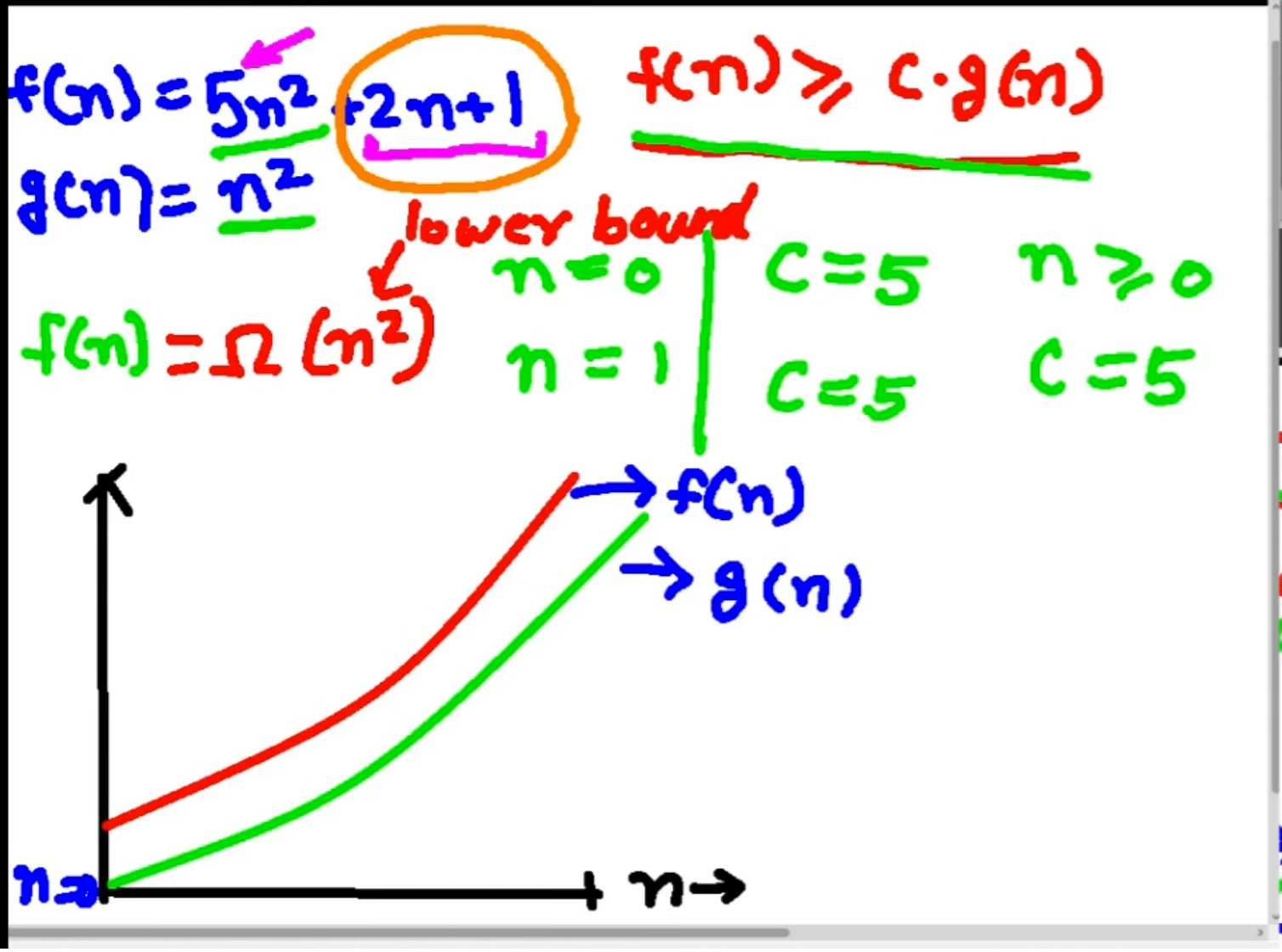“Omega” notation

“Theta” notation, Θ The notation Θ(n) is the formal way to express both the lower bound and the upper bound of an algorithm’s running time. Also known as tight bound. It is written mathematically as below-

``````Θ(g(n) = {f(n) : there exists c1, c2 and n0 such that c1.g(n) <= f(n) <= c2.g(n) for all n >= n0 }
``````

We will consider the diagram to understand it. As you can see we need the value of constant to satisfy the condition. For f(n)≤ c1.g(n), we will take the value of c1 as 8. This is similar to “Big Oh” notation. For f(n)≤c2.g(n), we will take the value of c2 as 5. This is similar to “Omega” notation. Now, when we plot the graph it is a combination of “Big Oh” and “Omega” notation.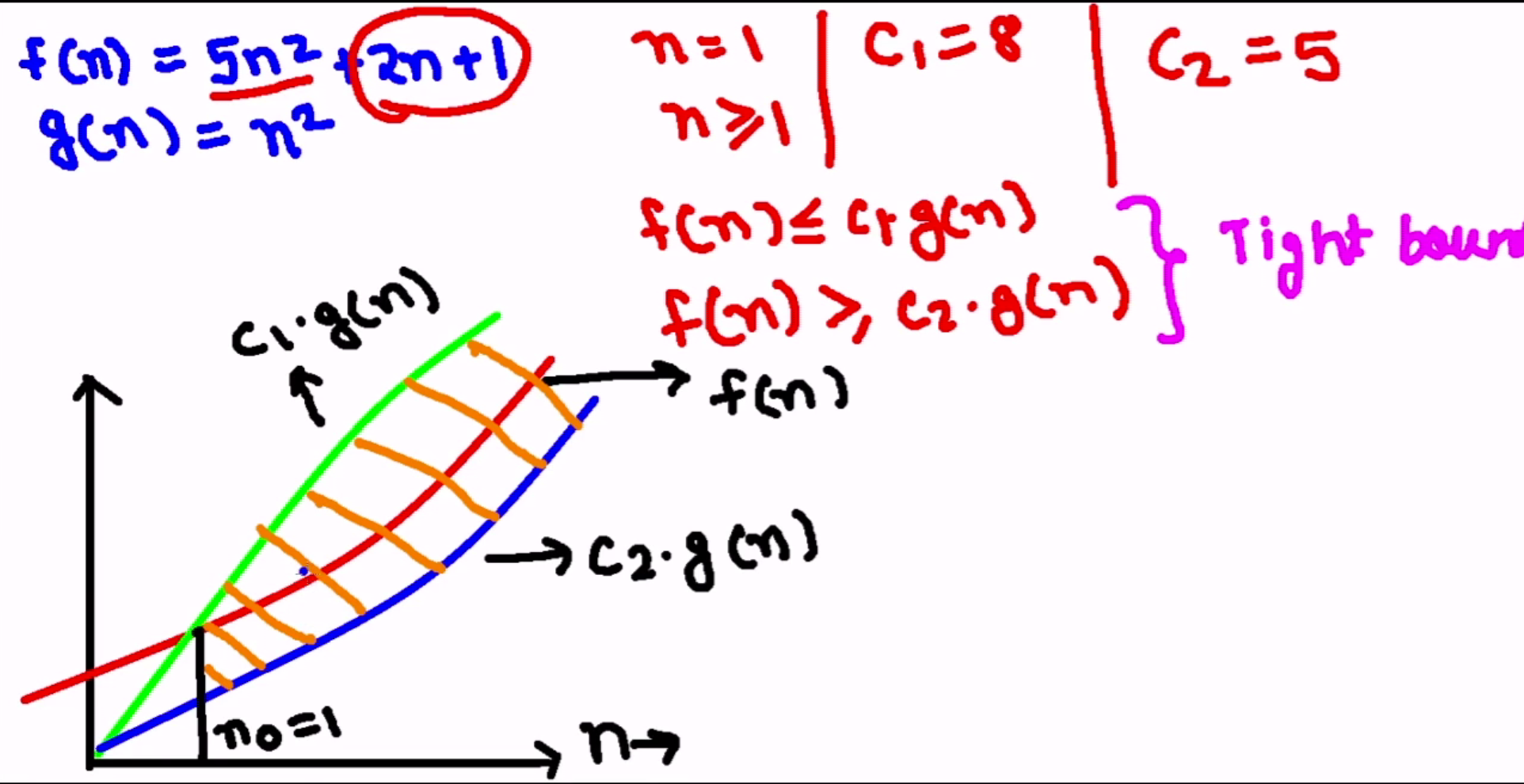“Theta” notation

This concludes the part-12 of the series and concludes Algorithm running time questions.

This post is also available on DEV.